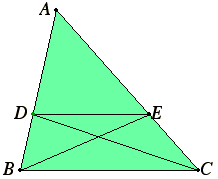# Proposition 2

If a straight line is drawn parallel to one of the sides of a triangle, then it cuts the sides of the triangle proportionally; and, if the sides of the triangle are cut proportionally, then the line joining the points of section is parallel to the remaining side of the triangle.

Let DE be drawn parallel to BC, one of the sides of the triangle ABC.

I say that BD is to AD as CE is to AE.Join BE and CD.

I.37

Therefore the triangle BDE equals the triangle CDE, for they are on the same base DE and in the same parallels DE and BC.

And ADE is another triangle.

V.7

But equals have the same ratio to the same, therefore the triangle BDE is to the triangle ADE as the triangle CDE is to the triangle ADE.

VI.1

But the triangle BDE is to ADE as BD is to AD, for, being under the same height, the perpendicular drawn from E to AB, they are to one another as their bases.

For the same reason, the triangle CDE is to ADE as CE is to AE.

V.11

Therefore BD is to AD also as CE is to AE.

Next, let the sides AB and AC of the triangle ABC be cut proportionally, so that BD is to AD as CE is to AE. Join DE.

I say that DE is parallel to BC.

With the same construction, since BD is to AD as CE is to AE, but BD is to AD as the triangle BDE is to the triangle ADE, and CE is to AE as the triangle CDE is to the triangle ADE, therefore the triangle BDE is to the triangle ADE as the triangle CDE is to the triangle ADE.

Therefore each of the triangles BDE and CDE has the same ratio to ADE.

V.9

Therefore the triangle BDE equals the triangle CDE, and they are on the same base DE.

I.39

But equal triangles which are on the same base are also in the same parallels.

Therefore DE is parallel to BC.

Therefore, if a straight line is drawn parallel to one of the sides of a triangle, then it cuts the sides of the triangle proportionally; and, if the sides of the triangle are cut proportionally, then the line joining the points of section is parallel to the remaining side of the triangle.

Q.E.D.

## Guide

Euclid prefers to prove a pair of converses in two stages, but in some propositions, as this one, the proofs in the two stages are almost inverses of each other, so both could be proved at once.

In this proposition we have a given triangle ABC and a line DE joining a point D on the side BC to a point E on the side AC. The claim is that

BD : AD = CE : AE if and only if DE || BC.

By the previous proposition VI.1 we know in any case that

BD : AD = triangle BDE : triangle ADE, and
CE : AE = triangle CDE : triangle ADE.

Hence,

BD : AD = CE : AE if and only if BDE : ADE = CDE : ADE.

By propositions V.7 and V.9 the latter condition is equivalent to BDE = CDE, and that, in turn, by propositions I.37 and I.39 is equivalent to DE || BC.

#### Note

It should be noted that a proportion such as BD : AD = AE : CE is not intended. In that case the sides are cut proportionally, but the correspondence is not the intended one.

#### Use of this theorem

This proposition is frequently used in the rest of Book VI starting with the next proposition. It is also used in Books XI and XII.